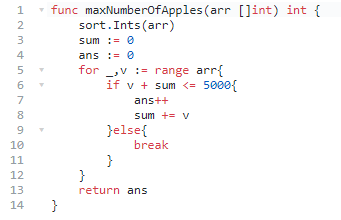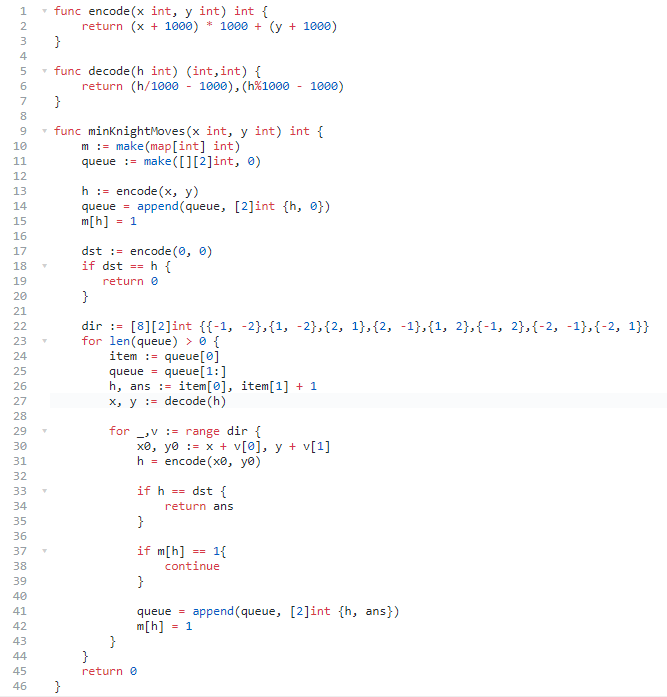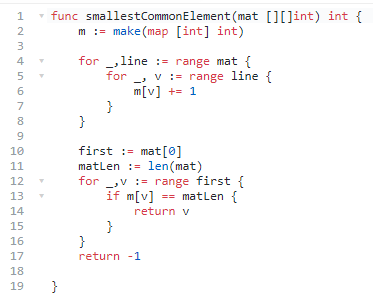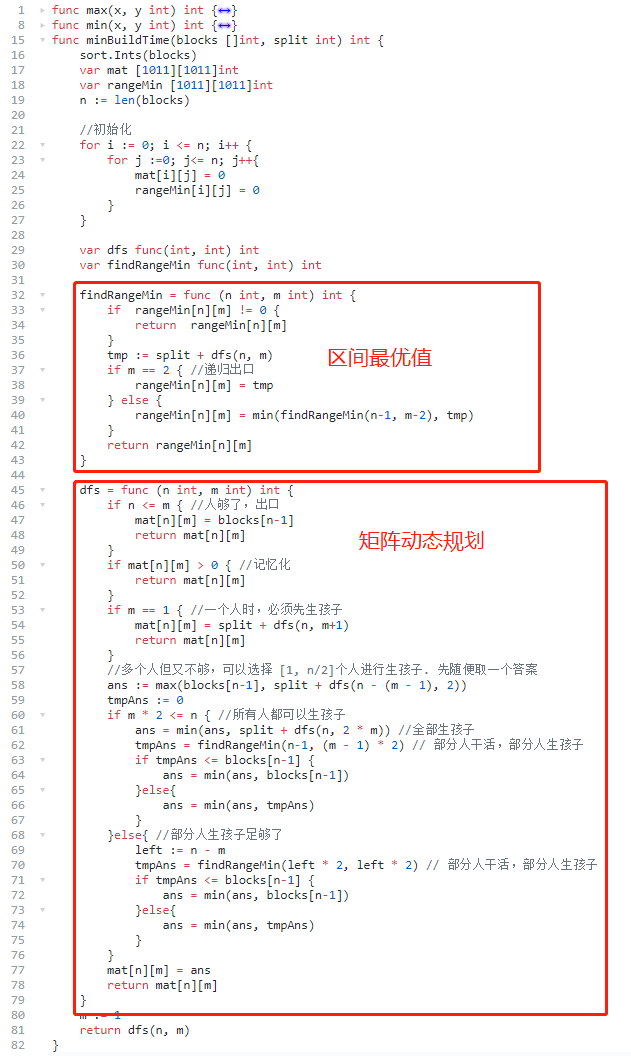# Leetcode 第9场双周赛回顾

## 一、最多可以买到的苹果数量## 二、进击的骑士## 三、找出所有行中最小公共元素## 四、建造街区的最短时间

``````0 个人干活，m 个人生孩子，剩余建筑 n 个。
1 个人干活，m-1 个人生孩子，剩余建筑 n -1 个。
...
m-1 个人干活， 1 个人生孩子， 剩余建筑 n - m + 1 个。
``````

``````f(n, m) = min( F(n, m, i) )
0 <= i < m
``````

`i`个人干活时，他们的耗时是固定的，即木桶短板理论，干活最慢的耗时就是总耗时。

``````F(n, m, i) = max(Data[n] , f(n - i,  2 * (m - i)))
``````

``````i=0, f(n, 2 * m)
i=1, f(n - 1, 2 * (m - 1))
i=2, f(n - 2, 2 * (m - 2))
...
i=m-1, f(n - (m - 1), 2)
``````

``````M(n, m) =  min(f(n, 2m), M(n - 1, m - 1))
``````## 五、最后

-EOF-

QQ算法群：165531769（不止算法）

## 关注公众号,接收最新消息

1.## 关注小密圈,学习各种算法

1.